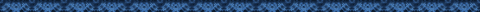Maryland School Performance Program
Mathematics
High School Core Learning Goals

 Goal 1: Functions and Algebra The student will demonstrate the ability to investigate, interpret, and communicate solutions to mathematical and real-world problems using patterns, functions, and algebra. Goal 2: Geometry, Measurement, and Reasoning The student will demonstrate the ability to solve mathematical and real-world problems using measurement and geometric models and will justify solutions and explain processes used. Goal 3: Data Analysis and Probability The student will demonstrate the ability to apply probability and statistical methods for representing and interpreting data and communicating results, using technology when needed. 1.1. Expectation: The student will analyze a wide variety of patterns and functional relationships using the language of mathematics and appropriate technology. 2.1. Expectation: The student will represent and analyze two- and three-dimensional figures using tools and technology when appropriate. 3.1. Expectation: The student will collect, organize, analyze, and present data. 1.1.1 The student will recognize, describe, and extend patterns and functional relationships that are expressed numerically, algebraically, and geometrically. 2.1.1 The student will describe the characteristics of geometric figures and will construct or draw geometric figures using technology and tools. 3.1.1 The student will design and/or conduct an investigation that uses statistical methods to analyze data and communicate results. 1.1.2 The student will represent patterns and functional relationships in a table, as a graph, and/or by mathematical expression. 2.1.2 The student will identify and verify properties of geometric figures using concepts from algebra and using the coordinate plane. 3.1.2 The student will use the measures of central tendency and variability to make informed conclusions. 1.1.3 The student will add, subtract, multiply, and divide algebraic expressions. 2.1.3 The student will use transformations to move figures, create designs, and demonstrate geometric properties. 3.1.3 The student will use simulations to make statistical inferences from data to estimate the probability of an event. 1.1.4 The student will describe the graph of a non-linear function in terms of the basic concepts of maxima and minima, roots, limits, rate of change, and continuity. 2.1.4 The student will validate properties of geometric figures using appropriate tools and technology. 1.2 Expectation: The student will model and interpret real-world situations, using the language of mathematics and appropriate technology. 2.2 Expectation: The student will apply geometric properties and relationships to solve problems using tools and technology when appropriate. 3.2 Expectation: The student will apply the basic concepts of statistics and probability to predict possible outcomes of real-world situations. 1.2.1 The student will determine the equation for a line, solve linear equations, and describe the solutions using numbers, symbols, and graphs. 2.2.1 The student will identify and verify congruent and similar figures and apply equality or proportionality of their corresponding parts. 3.2.1 The student will make informed decisions and predictions based upon the results of simulations and data from research. 1.2.2 The student will solve linear inequalities and describe the solutions using numbers, symbols, and graphs. 2.2.2 The student will solve problems using two-dimentional figures and/or right triangle trigonometry. 3.2.2 The student will make predictions by finding and using a line of best fit and by using a given curve of best fit. 1.2.3 The student will solve and describe if and where two straight lines intersect using numbers, symbols, and graphs. 2.2.3 The student will identify anduse inductive and deductive reasoning. 3.2.3 The student will communicate the use and misuse of statistics. 1.2.4 The student will describe how the graphical model of a non-linear function represents a given problem and will estimate the solution. 2.3 Expectation: The student will apply concepts of measurements using tools and technology when appropriate. 1.2.5 The student will apply formulas and use matrices to solve real-world problems. 2.3.1 The student will use algebraic and geometric properties to measure indirectly. 2.3.2 The student will use techniques of measurement and will estimate, calculate, and compare perimeter, circumference, area, volume, and surface area of two- and three-dimensional figures and their parts. The results will be expressed with appropriate precision.